/

### Mixtures of normal distributions

1.            Suppose we have two Normal distributions,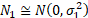and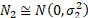, with the same mean (which without loss of generality is set to zero) but with different standard deviations. Suppose that we form a mixture,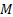, of these two different distributions, i.e. a distribution where the probability of drawing from the first distribution is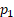and from the second distribution is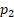where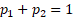. The probability density functions of the two distributions in isolation and of the mixture are: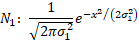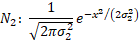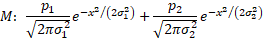2.            The (excess) kurtosis of the mixture is as follows: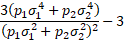3.            If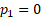or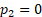or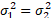then the mixture simplifies to a single normal distribution and thus has (excess) kurtosis of zero. However in other situations the excess kurtosis is greater than zero. This corresponds to the observation that the weighted arithmetic average of different positive numbers is greater than their geometric average, as long as more than one is given a positive weight.

4.            More generally, if we have a mixture of several different normal distributions (e.g. we postulate that we are in the presence of time-varying volatility and we are measuring time averaged statistics), and if each of these normal distributions has the same underlying mean, then we can expect our time averaged probability distribution to appear to exhibit excess kurtosis.

5.            If the distributions have different means then the magnitude of the excess kurtosis also depends on the means. In the above example, if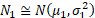and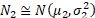then the probability density of the mixture becomes: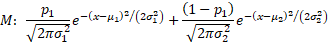and its excess kurtosis becomes: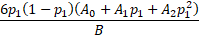6.            If the means are different enough (relative to differences in the standard deviations then the excess kurtosis can be negative, e.g. the excess kurtosis of an equal mixture (i.e.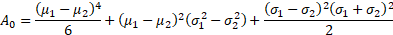) of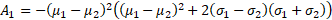and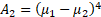has an excess kurtosis of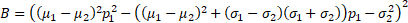. This is consistent with the observation that if the standard deviations of the every component element of the mixture are arbitrarily small relative to the spread of the means then any probability density function can be approximated arbitrarily accurately by giving suitable weights to different elements of the mixture, as long as there are enough suitably spaced contributors to the mixture. The weight to give to the element with mean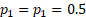in this formulation is the probability density at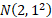of the desired overall probability distribution,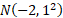.